• 今天小编就为大家分享一篇PyTorch 普通卷积和空洞卷积实例，具有很好的参考价值，希望对大家有所帮助。一起跟随小编过来看看吧
• conv2 = nn.Conv2d(1, 1, 3, stride=1, bias=False, dilation=2) # dilation就是空洞率，即间隔 init.constant_(conv1.weight, 1) init.constant_(conv2.weight, 1) out1 = conv1(arr) out2 = conv2(arr) print('...
from torch import nn
import torch.nn.init as init
def transform():
return Compose([
ToTensor(),
# Normalize((12,12,12),std = (1,1,1)),
])

arr = range(1,26)
arr = np.reshape(arr,[5,5])
arr = np.expand_dims(arr,2)
arr = arr.astype(np.float32)
# arr = arr.repeat(3,2)
print(arr.shape)
arr = transform()(arr)
arr = arr.unsqueeze(0)
print(arr)

conv1 = nn.Conv2d(1, 1, 3, stride=1, bias=False, dilation=1)  # 普通卷积
conv2 = nn.Conv2d(1, 1, 3, stride=1, bias=False, dilation=2)  # dilation就是空洞率，即间隔
init.constant_(conv1.weight, 1)
init.constant_(conv2.weight, 1)
out1 = conv1(arr)
out2 = conv2(arr)
print('standare conv:\n', out1.detach().numpy())
print('dilated conv:\n', out2.detach().numpy())

输出：
(5, 5, 1)
tensor([[[[ 1., 2., 3., 4., 5.],
[ 6., 7., 8., 9., 10.],
[11., 12., 13., 14., 15.],
[16., 17., 18., 19., 20.],
[21., 22., 23., 24., 25.]]]])
standare conv:
[[[[ 63. 72. 81.]
[108. 117. 126.]
[153. 162. 171.]]]]
dilated conv:
[[[[117.]]]]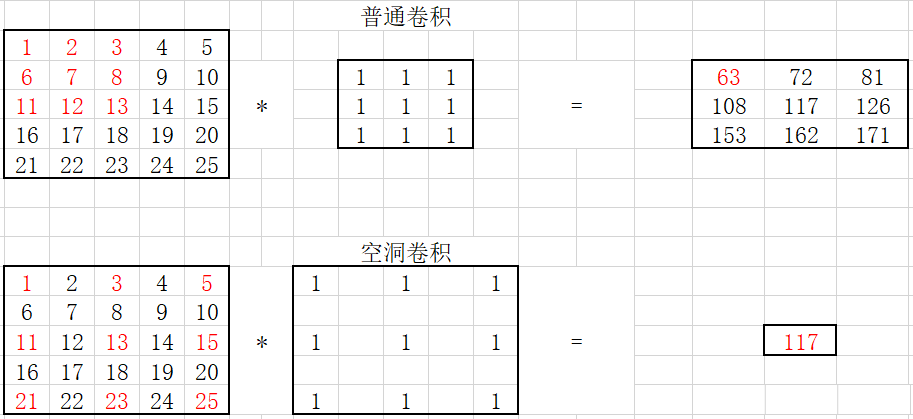转自：https://blog.csdn.net/hiudawn/article/details/84500648
展开全文• 1.空洞卷积 注要内容如下： 空洞卷积理解 但是呢，对于这篇博客的部分内容我不赞同。 3x3的kernel设置dialted-rate=2时，理应变为"5x5"的kernel，多出来的空洞用0填充，这也是变相的增加了感受野。也就是说，设置...
1.空洞卷积
主要内容如下： 空洞卷积理解 但是呢，对于这篇博客的部分内容我不赞同。 3x3的kernel设置dialted-rate=2时，理应变为"5x5"的kernel，多出来的空洞用0填充，这也是变相的增加了感受野。也就是说，设置dialted-rate>1时，会使得卷积的kernel变大。
2.代码验证
import numpy as np
import torch
import torch.nn as nn

arr = np.array(range(1,26))
arr = arr.reshape((5,5))

# 增加一个dim，为通道。
arr = np.expand_dims(arr,2)
print(arr.shape) # (5,5,1)

# piillow或者numpy 必须转换成tensor的格式
# h*w*c -> c*h*w
arr = arr.transpose((2,0,1))

print(arr.shape) # (1,5,5)
arr = torch.Tensor(arr)
print(arr)

# 增加一个fake batch dimension
arr = arr.unsqueeze(0)
print(arr.size()) #torch.Size([1, 1, 5, 5])

conv1 = nn.Conv2d(1, 1, 3, stride=1, bias=False, dilation=1)  # 普通卷积
conv2 = nn.Conv2d(1, 1, 3, stride=1, bias=False, dilation=2)  # dilation就是空洞率，间隔为2.多出来的用0填充

# 给参数初始化为1，方便运算
nn.init.constant_(conv1.weight, 1)
nn.init.constant_(conv2.weight, 1)

out1 = conv1(arr)
out2 = conv2(arr)

# Can't call numpy() on Tensor that requires grad. Use tensor.detach().numpy() instead.
print('standare conv:\n', out1.data.numpy())
print('dilated conv:\n', out2.data.numpy())

最终都是四维的输出
standare conv:
[[[[ 63.  72.  81.]
[108. 117. 126.]
[153. 162. 171.]]]]
dilated conv:
[[[[117.]]]]

两组卷积过程示意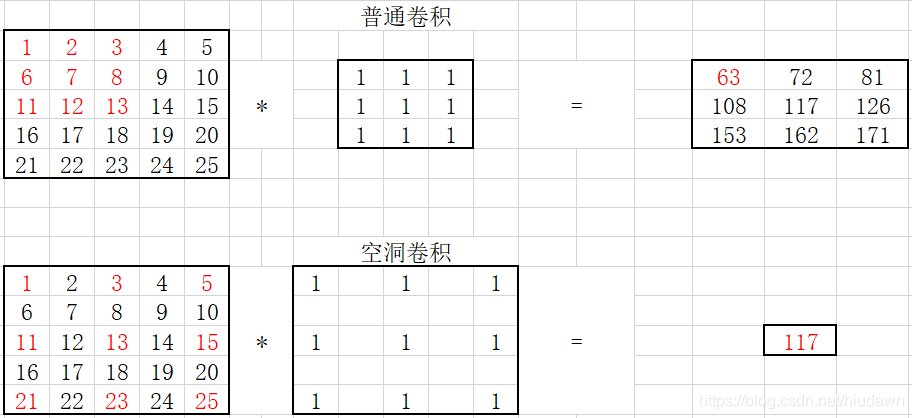图片来源
展开全文• 二维卷积实验 卷积神经网络是一种具有局部连接、权重共享特性的深层前馈神经网络。局部连接是指通过引入卷积核，使得每次运算学习的特征只和局部输入相关，极大地减少了计算量和连接层数；权值共享是指学习的特征...
Pytorch系列文章：

Pytorch实验一：从零实现Logistic回归和Softmax回归Pytorch实验2：手动实现前馈神经网络、Dropout、正则化、K折交叉验证，解决多分类、二分类、回归任务Pytorch实验3：动手实现卷积神经网络、空洞卷积、残差网络Pytorch实验4：手动/torch实现三种循环神经网络解决交通流量预测任务Pytorch实验5：疫情微博文本情感分类 (简化版SMP2020赛题)Pytorch实验6：论文代码中的高级语法

算法系列文章

算法1：遍历与枚举算法2：分而治之

大数据系列文章

目录：实现卷积神经网络、空洞卷积、残差网络
实验内容二维卷积实验空洞卷积实验残差网络实验
实验环境及实验数据集实验过程二维卷积实验手动实现二维卷积使用torch实现二维卷积对不同超参数的实验结果对比分析复现AlexNet模型与前馈神经网络的效果进行对比
空洞卷积实验残差网络实验
实验结果二维卷积实验手动实现二维卷积使用torch实现二维卷积对不同超参数的实验结果对比分析卷积核大小的影响卷积层数的影响
复现AlexNet模型与前馈神经网络的效果进行对比
空洞卷积实验空洞卷积实验结果空洞卷积与普通卷积的效果对比超参数的对比卷积核大小的影响卷积层数的影响

残差网络实验残差网络实验结果残差空洞卷积实验结果

实验心得体会参考文献写文不易 欢迎投喂！

实验内容
二维卷积实验
卷积神经网络是一种具有局部连接、权重共享特性的深层前馈神经网络。局部连接是指通过引入卷积核，使得每次运算学习的特征只和局部输入相关，极大地减少了计算量和连接层数；权值共享是指学习的特征具有局部不变性，使得对此类特征提取更加充分。 在深度学习中，卷积的定义和分析数学、信号处理中的定义略有不同。一般地，卷积通过互相关(cross-correlation)计算。给定输入

X

∈

R

M

×

N

\textbf{X}\in R^{M\times N}

和卷积核

W

∈

R

U

×

V

\textbf{W}\in R^{U\times V}

，它们的互相关为

y

i

,

j

=

∑

u

=

1

U

∑

v

=

1

V

w

u

,

v

x

i

+

u

−

1

,

j

+

v

+

1

y_{i,j}= \sum_{u=1}^{U}{ \sum_{v=1}^{V}{w_{u,v}x_{i+u-1,j+v+1}}}

也记作：

Y

=

W

⊗

X

\textbf{Y}=\textbf{W}⊗\textbf{X}

F

F

)、卷积核大小(

K

K

)、步长(

S

S

)、填充(

P

P

)，和输入图片的通道数(

D

D

)、宽度(

W

W

)、高度(

H

H

)共同决定；其中输出的通道数、宽度、高度分别是：

D

o

u

t

=

F

D_{out}=F

W

o

u

t

=

W

+

2

P

−

K

S

+

1

W_{out}=\frac{W+2P-K}{S}+1

H

o

u

t

=

H

+

2

P

−

K

S

+

1

H_{out}=\frac{H+2P-K}{S}+1

对于每个卷积核，共引入

K

×

K

×

D

+

1

K\times K\ \times D\ +1

个参数；由于有F个卷积核，所以共有

F

×

D

×

K

2

+

F

F\times D\times K^2+F

个参数。 卷积层(Convolution Layer)通常与汇聚层(Pooling Layer)混合使用。汇聚层是一种下采样(down sample)操作，作用是减少参数和特征的数量、加快运算速度和增加模型的鲁棒性。常用的汇聚包括：最大汇聚和平均汇聚。 二维卷积实验的目的是：（1）手动实现二维卷积；（2）使用torch实现二维卷积；（3）进行实验结果的分析、超参数的对比分析；（4）复现AlexNet模型；（5）与前馈神经网络的效果进行对比。
空洞卷积实验
空洞卷积是指通过在卷积核的元素间插入空隙来增加感受野的方法。对于大小为

K

×

K

K\times K

的卷积核，在每两个元素中插入

D

−

1

D-1

个元素，则空洞卷积核的有效大小是：

K

′

=

K

+

(

K

−

1

)

×

(

D

−

1

)

K'=K+(K-1)×(D-1)

其中：D是空洞卷积的膨胀率。例如：原卷积核大小为

3

×

3

3\times3

，则空洞卷积核的单边大小为

3

+

(

3

−

1

)

×

(

2

−

1

)

=

5

3+\left(3-1\right)\times\left(2-1\right)=5

。普通卷积可以视为

D

=

1

D=1

时的空洞卷积。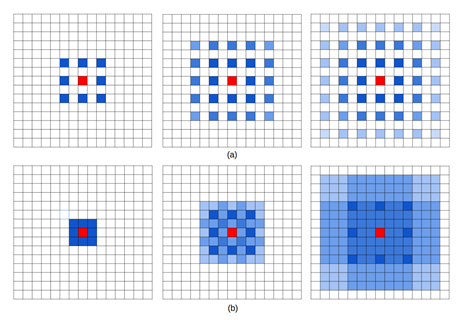由于空洞卷积引入了空隙，有一部分特征没有参与计算，且捕获具有一定间隔的特征可能是不相关的。Panqu Wang等人在2018年提出混合空洞卷积条件（Hybrid Dilated Convolution, HDC），对网络中每一层的膨胀率变化规律做出规定。满足此条件的网络可以规避特征空隙、同时捕获远程和近距离信息。 对于卷积核大小均为

K

×

K

K\times K

的N层卷积神经网络，其每一层膨胀率组成的序列分别为：

[

r

1

,

…

,

r

i

,

…

,

r

n

]

\left[r_1,\ldots,r_i,\ldots,r_n\right]

，需满足:

∀

1

<

i

<

n

,

M

i

≤

K

∀1<i<n,M_i≤K

其中：

M

i

=

m

a

x

[

r

i

+

1

−

2

r

i

,

2

r

i

−

r

i

+

1

,

r

i

]

Mi=max[r_{i+1}-2r_i,2r_i-r_{i+1},r_i]

本实验的目的是：（1）利用torch.nn实现空洞卷积，其中：膨胀率序列(dilation)满足HDC条件，在车辆分类数据集上实验并输出结果；（2）比较空洞卷积和普通卷积的结果；（3）选取1-2个超参数进行对比分析。
残差网络实验
在深度神经网络中，如果期望非线性单元

f

(

x

;

θ

)

f\left(\textbf{x};\theta\right)

去逼近目标函数

h

(

x

)

h(\textbf{x})

；可以考虑将目标函数分解为：

h

(

x

)

=

x

+

h

(

x

)

−

x

h(\textbf{x})=\textbf{x}+h(\textbf{x})-\textbf{x}

其中，

x

\textbf{x}

称为恒等函数(identity function)，

(

h

(

x

)

−

x

)

(h(\textbf{x})-\textbf{x})

称为残差函数(residue function)。 根据通用近似定理，非线性单元可以在理论上逼近任意函数。因此，原问题可以进行如下转化：期望

f

(

x

)

f\left(\textbf{x}\right)

逼近残差函数

(

h

(

x

)

−

x

)

(h(\textbf{x})-\textbf{x})

，使得

f

(

x

)

+

x

f\left(\textbf{x}\right)+\textbf{x}

逼近目标函数

h

(

x

)

h(\textbf{x})

。 一般地，残差网络可以通过跳层连接(shortcut connection)实现。过往的实验表明：残差网络可以解决深层神经网络的梯度爆炸、梯度消失和网络退化问题。 本实验的目的是：（1）复现给定的残差网络架构，分析结果；（2）结合空洞卷积，对比分析实验结果。
实验环境及实验数据集
数据集采用车辆分类数据集，共1358张车辆图片，三个类别。其中：随机抽取70%作训练集，30%作测试集。 实验环境为Linux 3.10.0-1062.el7.x86_64；运算器为NVIDIA GeForce RTX 2080；框架为：Pytorch 1.6.0；采用Pycharm内置的SSH连接进行交互。
实验过程
二维卷积实验
手动实现二维卷积
要实现卷积，需要依次实现：单通道互相关运算、多通道互相关运算、多卷积核互相关运算。需要说明的是，在Open CV读入的图片格式中，通道(channel)是最后一个维度，因此需要对读取的数据进行通道变换。代码段分别如下。
#单通道互相关运算
def corr2d(X,K):
batch_size,H,W = X.shape
h,w = K.shape,K.shape
Y = torch.zeros(batch_size,H-h+1,W-w+1).to(device)
for i in range(Y.shape):
for j in range(Y.shape):
area = X[:,i:i+h, j:j+w]
Y[:,i,j] = (area* K).sum()
return Y

#多通道互相关运算
def corr2d_multi_in(X, K):
res = corr2d(X[:,:,:,0],K[:,:,0])
for i in range(0,X.shape):
res += corr2d(X[:,:,:,i],K[:,:,i])
return res

#多卷积核互相关运算
def corr2d_multi_in_out(X, K):

定义了互相关运算、二维池化运算后，我们可以把卷积封装成卷积层模块。
#定义池化层
def pool2d(X, pool_size, mode='max'):
p_h, p_w = pool_size,pool_size
Y = torch.zeros((X.shape,X.shape,X.shape - p_h + 1, X.shape - p_w + 1))
for i in range(Y.shape):
for j in range(Y.shape):
if mode == 'max':
Y[:,:,i, j] = X[:,:,i: i + p_h, j: j + p_w].max()
elif mode == 'avg':
Y[:,:,i, j] = X[:,:,i: i + p_h, j: j + p_w].mean()
return Y

#定义卷积模块
class MyConv2D(nn.Module):
def __init__(self,in_channels,out_channels,kernel_size):
super(MyConv2D,self).__init__()
if isinstance(kernel_size,int):
kernel_size = (kernel_size,kernel_size)
self.weight = nn.Parameter(torch.randn((out_channels,in_channels)+kernel_size))
self.bias = nn.Parameter(torch.randn(out_channels,1,1))
def forward(self,x):
y = corr2d_multi_in_out(x,self.weight) +self.bias
return y


使用torch实现二维卷积
使用Pytorch可以直接定义模型完成训练。值得注意的是，由于卷积层的参数和输入图片大小会影响全连接层的参数设置，每一次调整网络架构时，全连接层的维度都需要重新计算。 此次实验中，我们把数据集中的图片压缩为200*100的尺寸，根据公式(3)~(5)，我们可以得出全连接层的维度是15532.
class ConvModule(nn.Module):
def __init__(self):
super(ConvModule,self).__init__()
self.conv = nn.Sequential(
nn.BatchNorm2d(32),
nn.ReLU(inplace=True)
)
self.pool = nn.MaxPool2d(2,2)
self.fc = nn.Linear(155232, num_classes)
def forward(self,X):
out = self.conv(X.float())
out = self.pool(out)
out = out.view(out.size(0), -1) # flatten each instance
out = self.fc(out)
return out

对不同超参数的实验结果对比分析
此部分，我们试验了不同的卷积层层数（在ConvModule中定义）和卷积核大小。
复现AlexNet模型
AlexNet中引入了Dropout、ReLU激活和局部相应归一化技巧，以提升模型的泛化能力。AlexNet中的局部响应归一化(Local Response Normalization, LRN)是一种对于同层的部分神经元的归一化方法。它受到生物学中侧抑制现象的启发，即：活跃神经元对邻近神经元由平衡和约束作用。数学上，可视作在激活函数的基础上，再做一次简单线性回归。此方法现已被池化层取代，很少应用。此处，我们直接采用中的LRN实现.
AlexNet是第一个现代深度网络模型，由Krizhevsky等人在2012年参与ImageNet竞赛中提出。由于当时GPU的运算性能限制，当时被拆分为两个子网络进行分别训练。它包含5个卷积层、3个汇聚层和3个全连接层。 在本实验中，我们主要参照中的AlexNet类进行实验。需要注意的是，多层网络、LRN、ReLU激活可能会带来大规模的梯度爆炸问题，造成损失为NaN。因此，我们在每一层卷积的末尾额外添加了一个BatchNorm层进行归一化处理。
与前馈神经网络的效果进行对比
我们将卷积网络与实验2中的网络（代码如下）进行对比。
layers = collections.OrderedDict([
('L1',nn.Linear(154587,192)),
('A1',nn.Hardswish()),
('drop1', nn.Dropout(p=0.1)),
('L2', nn.Linear(192, 96)),
('A2', nn.LeakyReLU()),
('drop2', nn.Dropout(p=0.1)),
('FC', nn.Linear(96,3))
])
AnnNet = nn.Sequential(layers)


空洞卷积实验
在torch中，定义空洞卷积较为简便，只需要修改卷积层的dilation参数即可。
nn.Conv2d(in_channels=3, out_channels=32, kernel_size=3, stride=1, padding=0, dilation=3) #膨胀率为3

残差网络实验由于复现的残差网络具有一定的子结构相似性，此处，我们进行了模块化处理。首先，我们定义两层卷积的残差网络：
class Residual(nn.Module):
def __init__(self, input_channels,num_channels, use_1x1conv=False, strides=1, **kwargs):
super(Residual,self).__init__()
if use_1x1conv:
self.conv3 = nn.Conv2d(num_channels,num_channels, kernel_size=1,stride=strides)
else:
self.conv3 = None
# AUTHOR:ZIXIN QIN
self.bn1 = nn.BatchNorm2d(num_channels)
self.bn2 = nn.BatchNorm2d(num_channels)

def forward(self, X):
Y = self.bn1(self.conv1(X))
Y = F.relu(Y)
Y = self.bn2(self.conv2(Y))
if self.conv3:
X = self.conv3(X)
return F.relu(Y + X)
return F.relu(Y)


之后，我们定义一层上采样、一层卷积的残差网络：
class UpsampleResidual(nn.Module):
def __init__(self, in_channel,out_channel, kernel_size=3,strides=1, **kwargs):
super(UpsampleResidual, self).__init__()
self.l1 = nn.Conv2d(in_channels=in_channel, out_channels=out_channel, kernel_size=kernel_size, stride=strides,padding=1)
self.shortcut = nn.Conv2d(in_channels=in_channel,out_channels=out_channel,kernel_size=1,stride=strides)
def forward(self,X):
Y = self.l1(X)
Y = F.relu(Y)
Y = self.l2(Y)
shortcut = self.shortcut(X)
return F.relu(Y+ shortcut)


最终，我们将以上两个模块进行多次复合，得到需要复现的网络：
class ResidualModule(nn.Module):
def __init__(self):
super(ResidualModule,self).__init__()
self.conv1 = nn.Conv2d(3,64,3)
self.conv2 = Residual(64,64,True)
self.conv3 = UpsampleResidual(64,128,3)
self.conv4 = Residual(128,128, True)
self.conv5 = UpsampleResidual(128,256,3)
self.conv6 = Residual(256,256, True)
self.conv7 = UpsampleResidual(256,512,3)
self.conv8 = Residual(512,512,True)
self.pool = torch.nn.AvgPool2d(kernel_size=3)
self.fc = nn.Linear(460800, num_classes)
self.process = nn.Sequential(self.conv1,self.conv2,self.conv3,
self.conv4,self.conv5,self.conv6,self.conv7,self.conv8)
def forward(self,X):
out = self.process(X)
out = out.view(out.size(0), -1) # flatten each instance
out = self.fc(out)
return out

实验结果
二维卷积实验
手动实现二维卷积
手动定义的卷积没有进行矩阵优化，时间复杂度为

O

(

n

4

)

\mathcal{O}\left(n^4\right)

，对运算时间要求较高。经过手动验证，反向传播过程耗费时间过长，无法预计完成模型训练的时间；因此，这里不能提供具体的手动实现的卷积的结果图表，但是可以观察到loss的计算过程。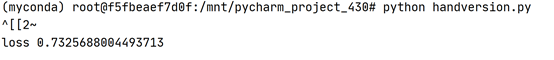使用torch实现二维卷积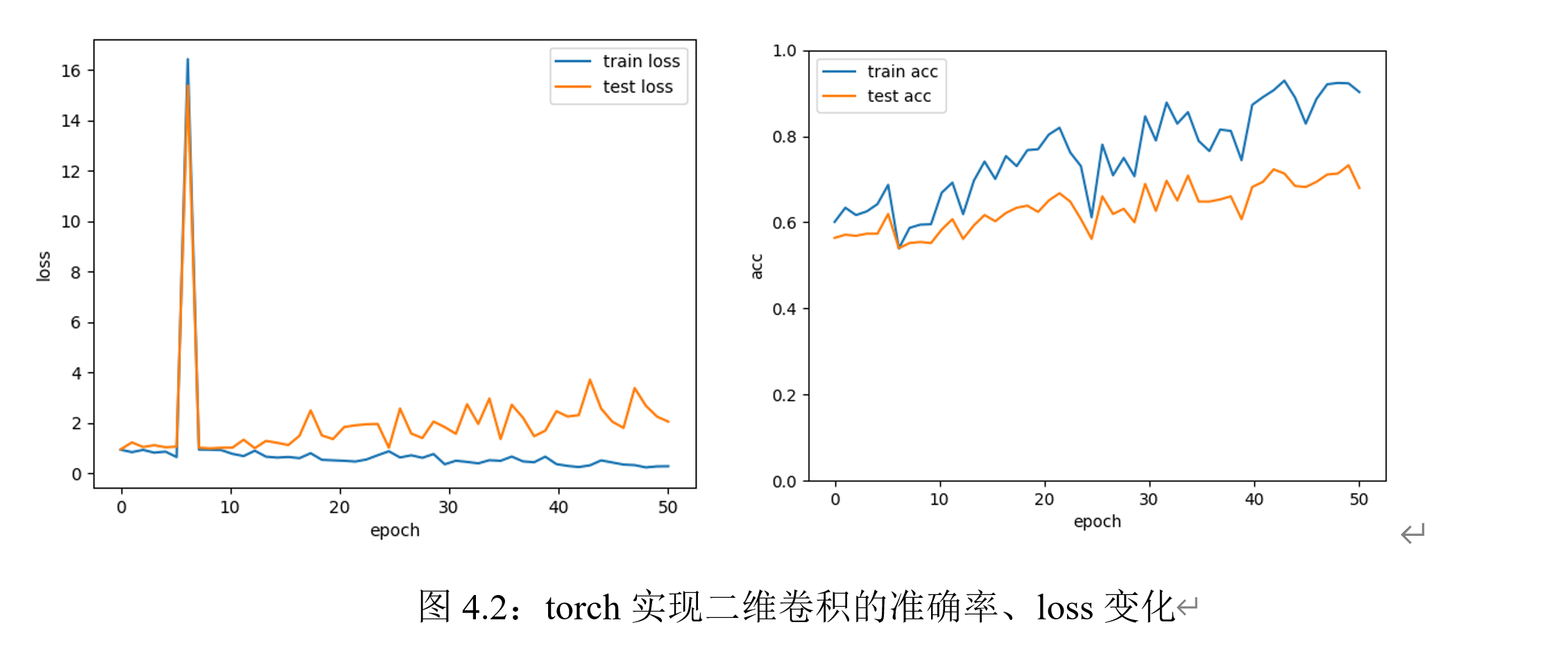实验迭代次数为50次时，在测试集、训练集上的loss和准确率变化如上图所示。可以观察到loss的震荡变化和准确率的逐渐上升；但训练集上的表现始终优于测试集，说明模型存在一定的过拟合现象。在GPU环境下，训练两层卷积神经网络模型训练50次epoch的时间为7分钟左右。
对不同超参数的实验结果对比分析
卷积核大小的影响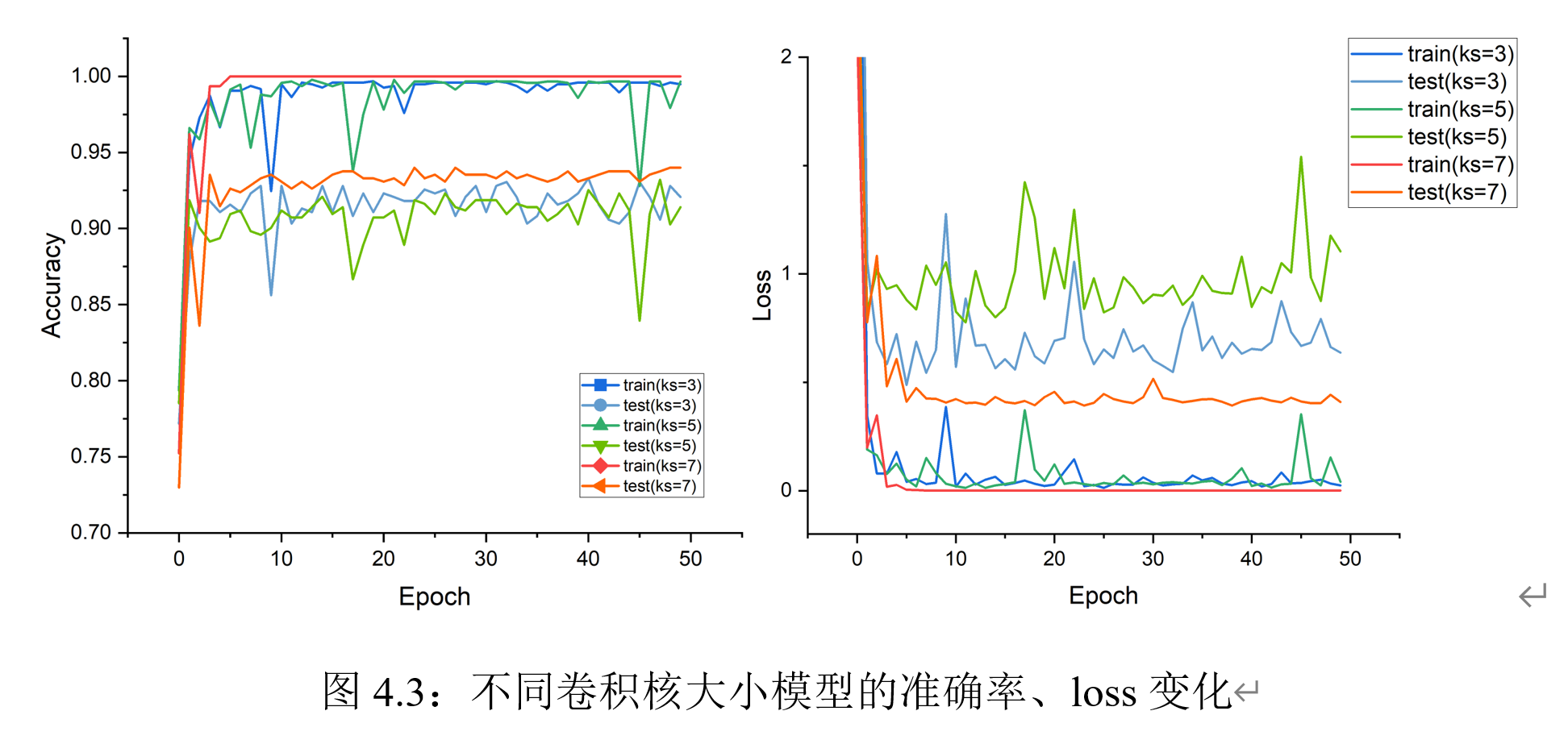可以观察到：尺寸相对较大的卷积核在训练时收敛更快、总体震荡较小，收敛时精度更高、loss更小。尺寸较小的卷积核在训练过程种震荡趋势明显。总体而言，不同尺寸卷积核的模型在此次训练时都存在着过拟合的趋势；可见，是否过拟合和卷积核大小无关。
卷积层数的影响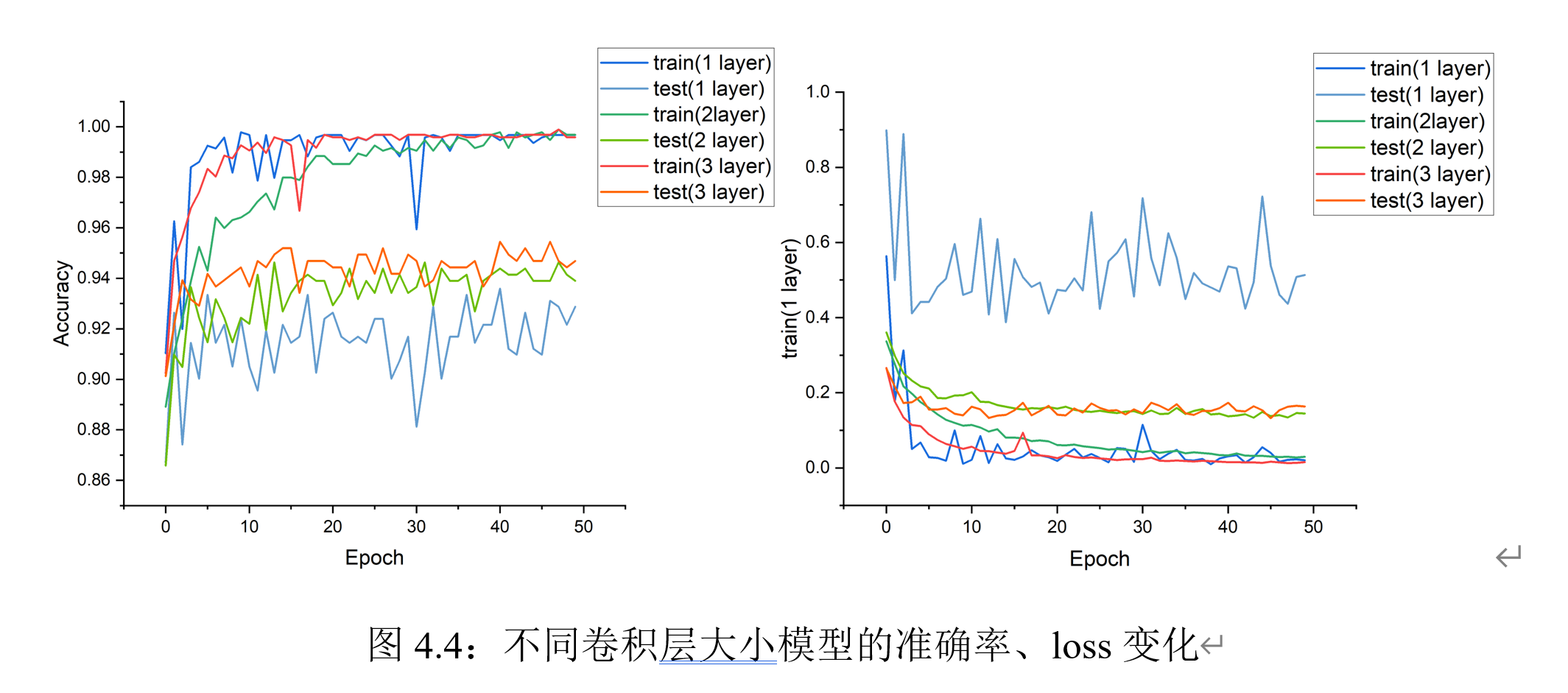可以观察到：随着卷积层数的增加：在训练阶段，训练集上的震荡更小；在收敛阶段，loss更低，在训练集上的准确率更高。在测试集上，三种网络的精确度表现近似，但卷积层数更多的网络相对收敛更快。
复现AlexNet模型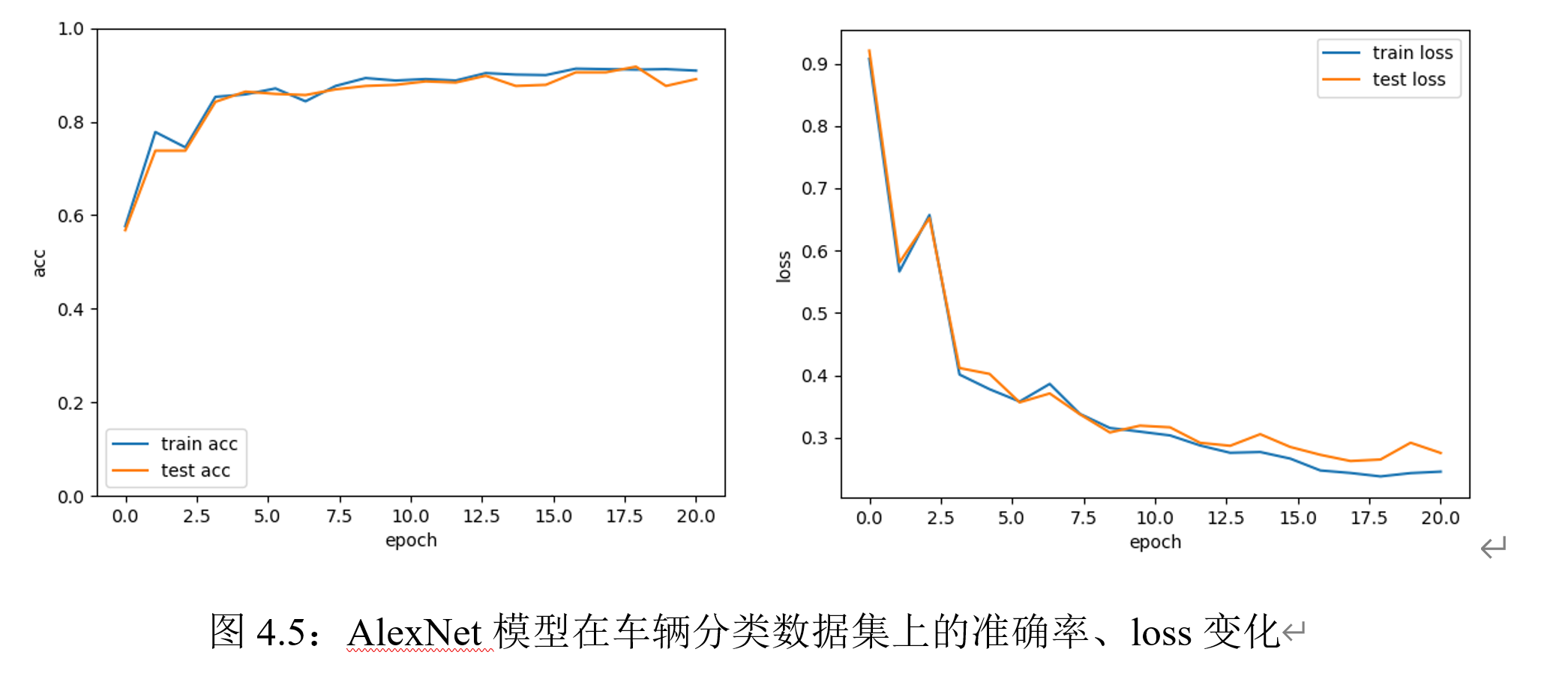在AlexNet上迭代50次的结果如上。可以观察到，随着层数的加深，网络收敛所需的epoch数明显增加。
与前馈神经网络的效果进行对比实验结果表明，在本实验所用的车辆分类数据集上，迭代20次时，前馈神经网络的效果适中，效果最优的是三层卷积神经网络。AlexNet在预测准确度的效果不佳，可能是原因是：（1）数据集过小，模型无法充分学习特征；（2）训练次数不够，模型没有收敛。但值得注意的是，AlexNet在测试集和训练集上的表现都非常接近，说明没有出现过拟合现象。
空洞卷积实验
空洞卷积实验结果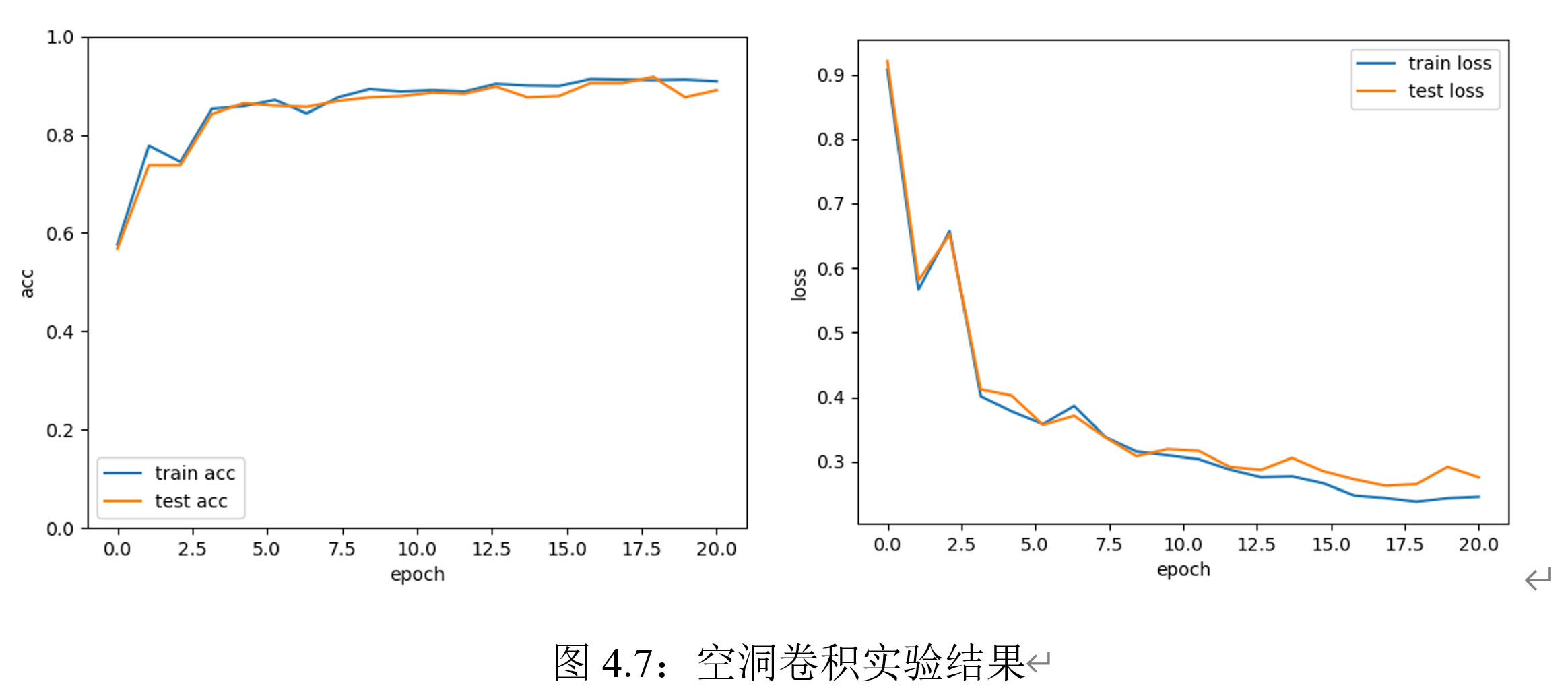空洞卷积的实验结果如上。可以观察到：相比于振荡现象明显的普通卷积，空洞卷积模型没有出现过拟合现象，可能是由于空洞卷积能很好地同时提取远近距离特征。
空洞卷积与普通卷积的效果对比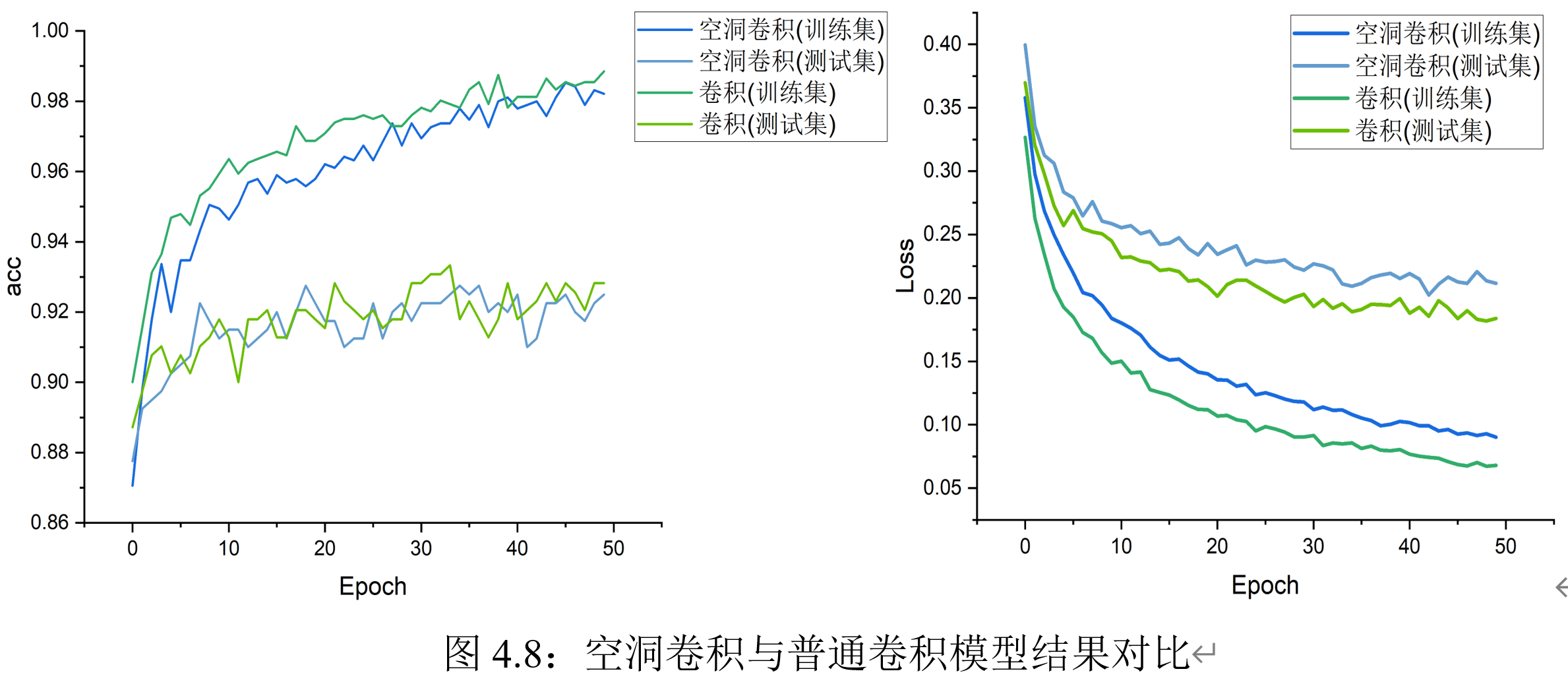从图像可以看出：空洞卷积的准确度变化更平稳、在测试集上的精度和普通卷积类似；在同样训练次数下，空洞卷积模型训练集上的精度略低于普通卷积。可能原因是：空洞卷积提取了更多的层次特征，需要更多的迭代次数才能收敛。 空洞卷积模型相比于普通卷积在时间上略有增加，每次训练平均实际在8分钟左右；但空洞卷积在不同超参数设定下，运行时间变化较大。经过实验验证，可以发现主要耗时的模块在于反向传播部分。
超参数的对比
卷积核大小的影响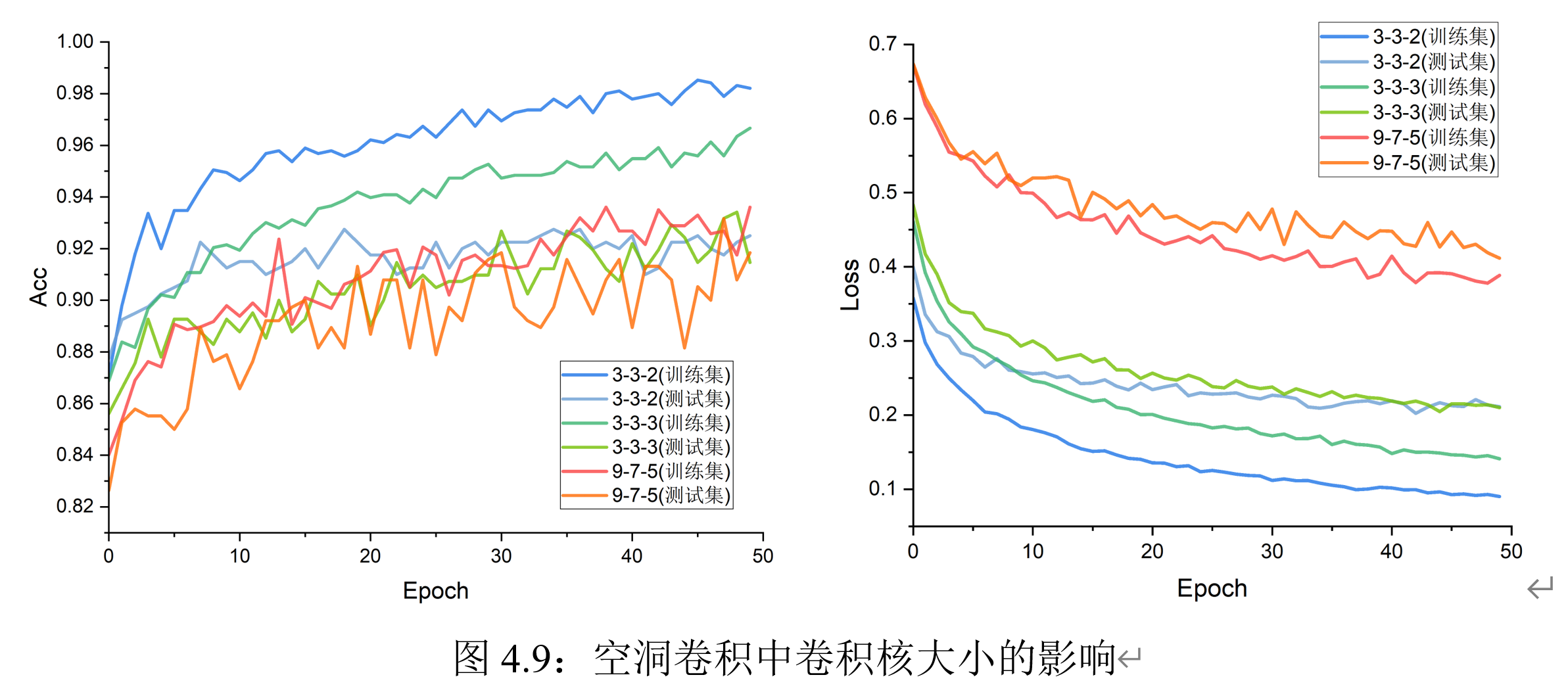实验结果表明：在空洞卷积模型训练前期，卷积核大小分别设置为3、3、2时在测试集上的表现较优、且变化趋势较稳定；在训练后期，模型逐渐收敛，卷积核大小对实验结果的影响减少。可能原因是：卷积核的尺寸增加等价于模型参数量增加，所以在训练前期可能会有更好的表达力。在空洞卷积中，尺寸大的卷积核并没有表现出普通卷积中出现的巨大性能提升。
卷积层数的影响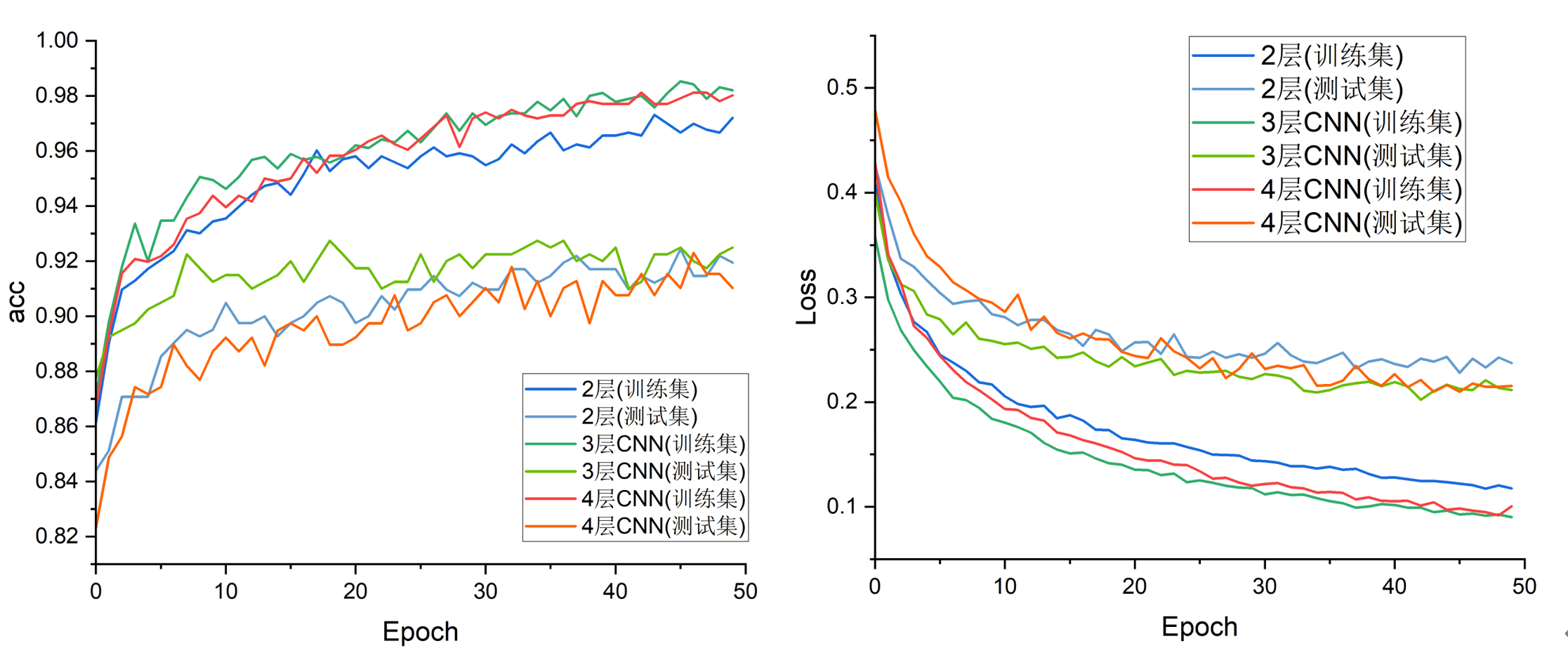可以观察到：在收敛阶段，深层的网络在训练集上准确度和loss上表现更好，但在测试集上没有突出的优势。
残差网络实验
残差网络实验结果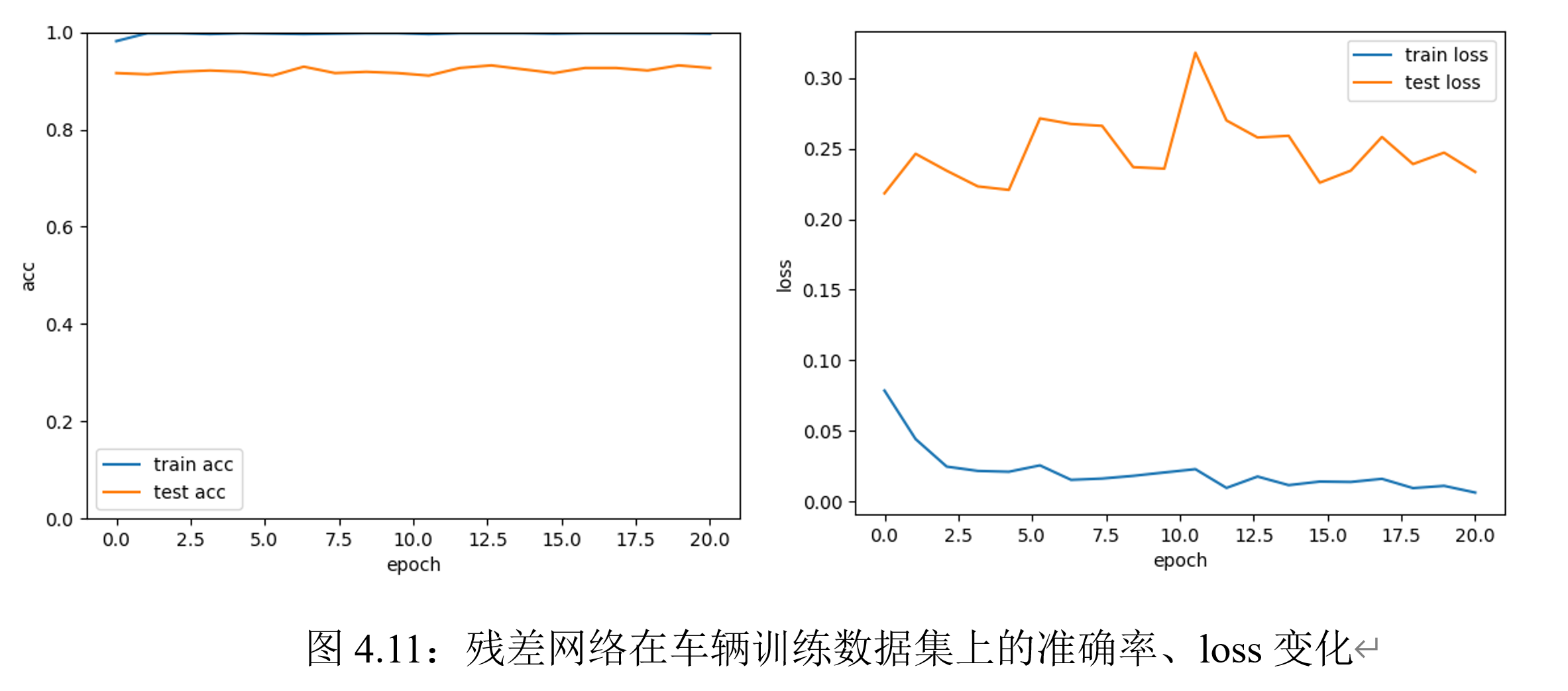观察实验结果可知：残差网络在训练集上迭代20次即可达到接近99%的准确率；此外，模型收敛速度非常快。可见，转化后的函数逼近问题更容易学习。
残差空洞卷积实验结果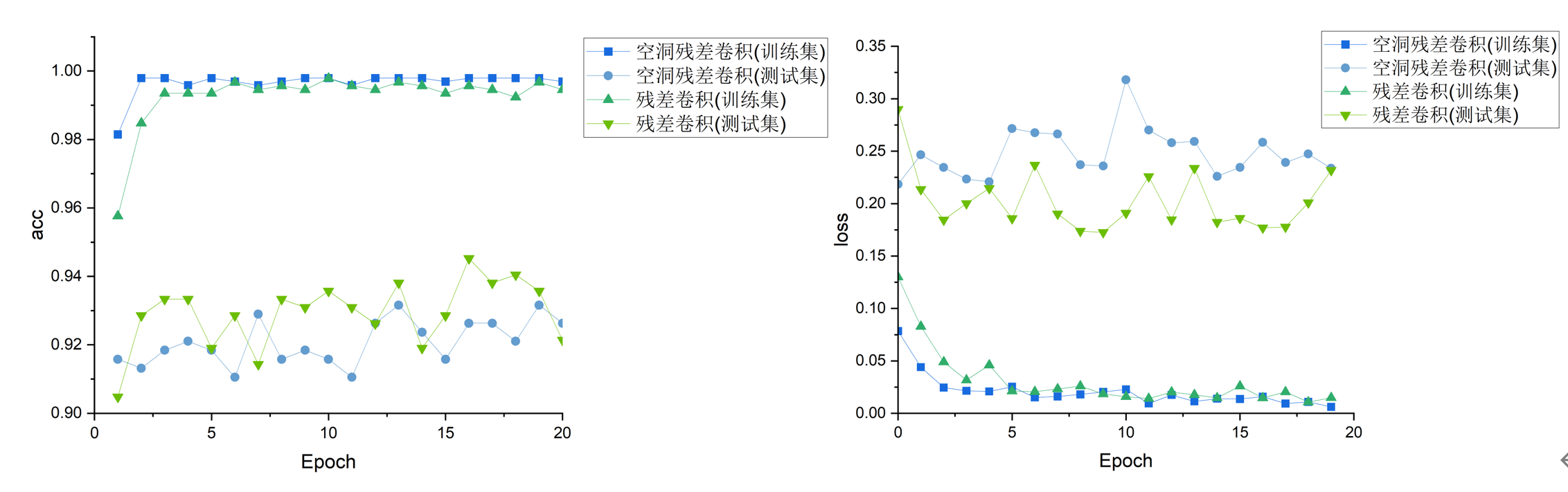实验结果显示，在训练集上，空洞残差卷积具有更高的精度；在测试集上，空洞残差卷积和普通残差卷积表现相似，两者均具有较高的精度。
实验心得体会
本次实验遇到的最大的问题是计算效率的问题；此外，在实验中，还有一些细节可以优化：：
进入卷积神经网络部分后，定义的网络在CPU上的训练时间已远远超出预期；因此，模型迁移到显存、使用GPU进行训练是必须的。在获取服务器使用权限后、进行模型训练后，我直观地体会到GPU带来的效率提升。手动实现的卷积时间复杂度太高，目前仍然无法使用。本实验主要使用torch的实现，没有采用手动卷积。如果后续有可能对此部分进行优化，需要对矩阵乘法部分进行Image to Column的并行化处理。数据集的生成。实验中采用的是OpenCV框架进行读取，但其实OpenCV的通道和RGB颜色的定义和torch中有较大的区别。因此，后续可以考虑使用其它的图片读取框架。超参数的导入。实验中每一层的超参数（卷积层数、卷积核通道数等）是固定的，但这不方便对超参数进行网格搜索。后续可以针对此问题，设置层数的传参。
参考文献
 Pytorch手撕经典网络之AlexNet. https://zhuanlan.zhihu.com/p/29786939  邱锡鹏，神经网络与深度学习，机械工业出版社，https://nndl.github.io/ , 2020.  Krizhevsky A, Sutskever I, Hinton G E. Wang P, Chen P, Yuan Y, et al. Understanding convolution for semantic segmentation[C]//2018 IEEE Winter Conference on Applications of Computer Vision (WACV). IEEE, 2018: 1451-1460.  Zhang, Aston and Lipton, Zachary C. and Li, Mu and Smola, Alexander J. Dive into Deep Learning
写文不易 欢迎投喂！展开全文深度学习 计算机视觉 神经网络
• 2016年提出的 空洞卷积广泛应用于语义分割与目标检测等任务中 空洞卷积(膨胀卷积/扩张卷积) Dilated/Atrous Convolution 空洞卷积是一种不增加参数数量同时增加输出单元感受野的一种方法。Atrous 卷积，就是带洞的...

2016年提出的

空洞卷积广泛应用于语义分割与目标检测等任务中
空洞卷积(膨胀卷积/扩张卷积) Dilated/Atrous Convolution
空洞卷积是一种不增加参数数量同时增加输出单元感受野的一种方法。Atrous 卷积，就是带洞的卷积，卷积核是稀疏的
空洞卷积的概念是从语义分割任务中发展出来的，是为了解决基于FCN思想的语义分割中，输出图像的size要求和输入图像的size一致而需要upsample，但由于FCN中使用pooling操作来增大感受野同时降低分辨率，导致upsample无法还原由于pooling导致的一些细节信息的损失的问题而提出的。为了减小这种损失，自然需要移除pooling层，因此空洞卷积应运而生。
原先的 CNN architecture 是用一个“实心”的 kernal 去扫描 input data，然后使用 pooling 方法直接暴力的删掉其余 75% 的信息量，只留下 25% 的原汁原味，这样的做法在还没有精确到 pixel-wise 的情况时还是可以行的通的，一旦要归类到小至 pixel 等级的尺度时，pooling 对原 data 的破坏力足以让事情搞砸，试想一个原始数据被经过 Convolution 剥离出了其区域特征后，接着下一个环节被大刀阔斧般的砍去内部信息，并且永久无法复原，小物体信息无法重建 (假设有四个pooling layer 则任何小于 2^4 = 16 pixel 的物体信息在理论上将无法重建和分割)。这是在微小的 pixel 世界中无法接受的做法。
如果说 pooling 这种简单删减 data 让单位 output 中 Receptive Field 增大的方法不可行，就需要一个新的在不删减原始数据的情况下，直接让 Receptive Field 提升的办法：Dilated Convolution。简单的说，就是把原本“实心”的 kernal 元素之间按照一定规律插入 0 元素作为空格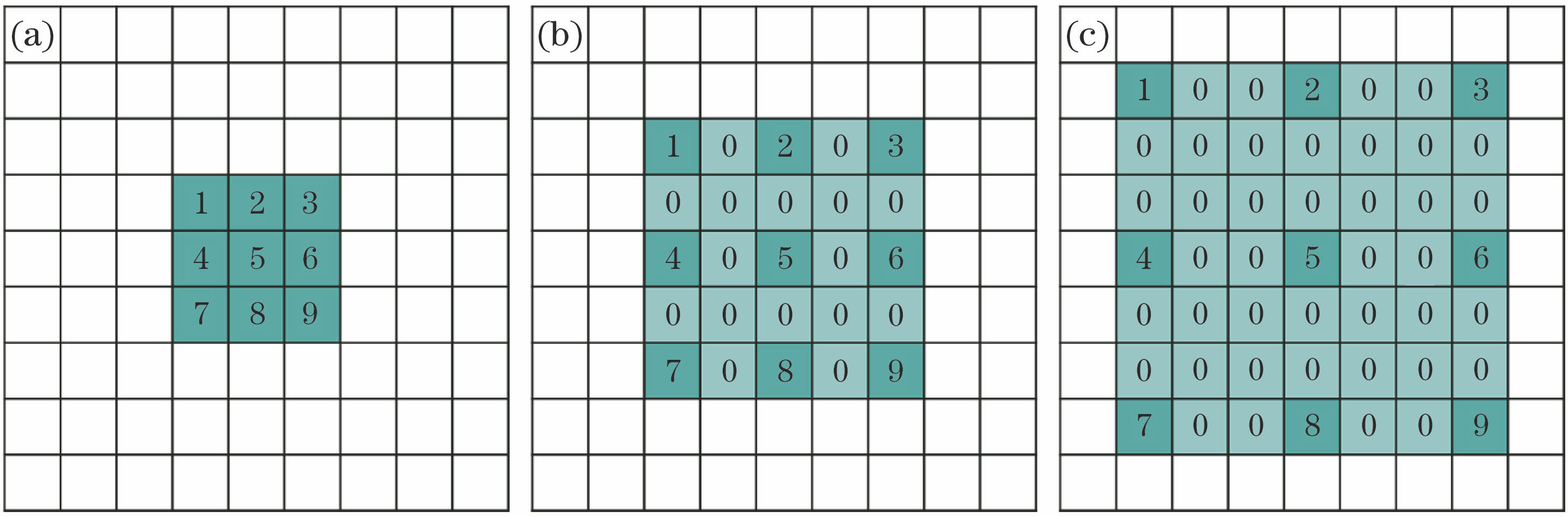空洞卷积向卷积层引入了一个称为 “扩张率(dilation rate)”的新参数，该参数定义了卷积核处理数据时各值的间距。
看下图可以对膨胀率有一个比较直观的理解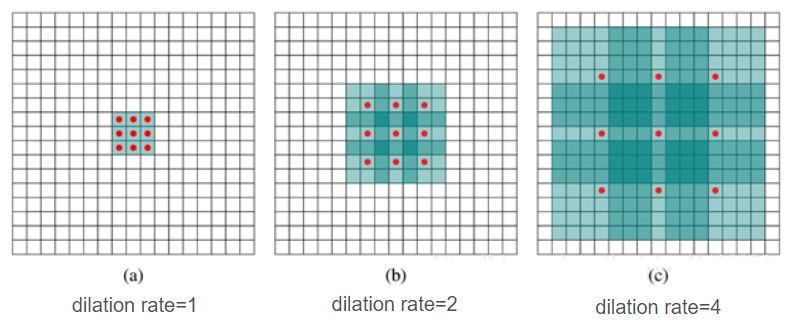假设原始特征为feat0
首先使用扩张率为1的空洞卷积生成feat1，feat1上一点相对feat0感受野为3*3（如图a）；
然后使用扩张率为2的空洞卷积处理feat1生成feat2（如图b），使第一次空洞卷积的卷积核大小等于第二次空洞卷积的一个像素点的感受野，图b即feat1上一个点综合了图a即feat0上3*3区域的信息，则生成的feat2感受野为7*7，即整个图b深色区域；
第三次处理同上，第二次空洞卷积的整个卷积核大小等于第三次空洞卷积的一个像素点的感受野，图c即feat2上每个点综合了feat0上7*7的信息（感受野），则采用扩张率为3的空洞卷积，生成的feat3每一个点感受野为15*15。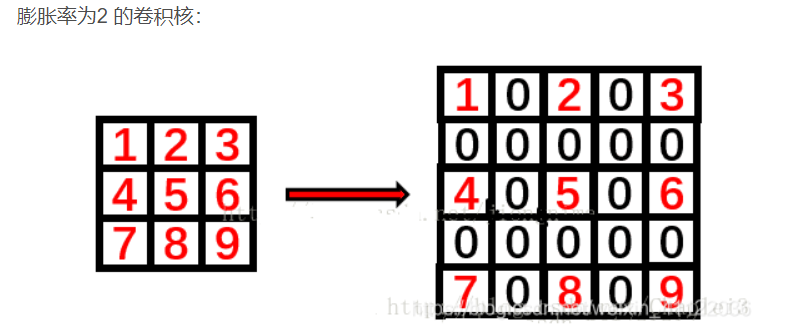展开全文语义分割
• Pytorch中空洞卷积分为两类，一类是正常图像的卷积，另一类是池化时候。 pytorch卷积API为： Conv2d(in_channels,out_...(1)正常图像空洞卷积： 感受野尺寸 size=(dilation-1)*(kernel_size-1) + kernel_size ...
• 博客见：https://blog.csdn.net/qq_37534947/article/details/109727232，主要是空洞卷积以及残差网络的代码实现，包含数据集，框架是pytorch
• 一：pytorch实现空洞卷积实验（torch实现） 要求： 从至少一个数据集上进行实验，同理，这里我选取了车辆分类数据集（后面的实验都是用的车辆分类数据集），主要在之前利用torch.nn实现二维卷积的基础上，为解决感受...
• 深度可分离卷积(Depthwise Separable Convolution)和分组卷积(Group Convolution)的理解，相互关系及PyTorch实现 1. 分组卷积(Group Convolution) 分组卷积最早出现在AlexNet中，如下图所示。在CNN发展初期，GPU资源...
• 放出来的训练代码，工作量应该不大，这个月应该就能做好，熟悉一下pytorch基础。 class Conv2d(nn.Module): def __init__(self, in_channels, out_channels, kernel_size, stride=1, same_padding=False, dilation=...
• 3、Rethinking Atrous Convolution for Semantic Image Segmentation(https://arxiv.org/abs/1706.05587) 4、书籍《深度学习之PyTorch物体检测实战》 5、如何理解空洞卷积（dilated convolution）？...网络 计算机视觉 人工智能 深度学习
• ASPP全称：Atrous ...另一个是Atrous，空洞卷积，两个加在一起就是ASPP。 首先说一下SPP：Spatial Pyramid Pooling。这个结构出自于论文《Spatial Pyramid Pooling in Deep Convolutional Networks for Visual ReSPP 语义分割
• 空间金字塔池化(Spatial Pyramid Pooling, SPP)原理及代码实现(Pytorch) 卷积神经网络(CNN)由卷积层和全连接层组成，其中卷积层对于输入数据的大小并没有要求，唯一对数据大小有要求的则是第一个全连接层，因此基本...python 算法
• 空洞卷积又叫虫洞卷积 在代码上面其实是一样的，就是参数改改数值而已 我们常见的卷积形式比如3*3的卷积核，在faeture map上，无间隔的累积和出新的faeture map。他的感受野大小是3 常见的代码写法： conv1 =...python 计算机视觉
• 我用pytorch实现了DUC功能，代码放在我的 github 上了，欢迎star，欢迎讨论。 DUC 语义分割任务下的网络基本都具有encoding和decoding的过程，而大多数网络在decoding时使用的是双线性插值。而双线性插值是不能学习...
• CNN从2012年的AlexNet发展至今，科学家们发明出各种各样的CNN模型，一个比一个深，一个比一个准确，一个比一个轻量。我下面会对近几年一些具有变革性的工作进行简单盘点，...Group convolution 分组卷积，最早在AlexN.神经网络 网络 机器学习 计算机视觉
• 文章目录分组卷积（Group Convolution）原理用途常规卷积（Convolution）空洞（扩张）卷积（Dilated/Atrous Convolution）深度可分离卷积（depthwise separable convolution）标准卷积与深度可分离卷积的不同深度可...
• 计算机视觉基本概念 灰度图即将c的通道变成1 当描述数据时，我们有时候只需要关注其纹理信息，就不需要关注其彩色空间。如果想要模型对于颜色的变化不那么敏感，我们在训练模型的时候就用灰度图...卷积运算：利用像
• 1、因果卷积 2、空洞因果卷积 3、HDC https://blog.csdn.net/qq_26369907/article/details/97624028 https://blog.csdn.net/suixinsuiyuan33/article/details/79451069
• TCN全称Temporal Convolutional Network，时序卷积网络，是在2018年提出的一个卷积模型，但是可以用来处理时间序列。 卷积如何处理时间序列 时间序列预测，最容易想到的就是那个马尔可夫模型： P(yk∣xk,xk−1,...,...网络 python 人工智能 深度学习
• 主要结合DeepLabv3+的网络结构和开源代码进行了对照解析。通过对网络结构中的每一块的作用及其代码实现中的细节进行了解，就能够对该网络模型有一个宏观和微观上的把握。DeepLabv3+ 网络结构 Decoder...